# Texas Go Math Grade 4 Lesson 8.4 Answer Key Multiply Using Partial Products

Refer to our Texas Go Math Grade 4 Answer Key Pdf to score good marks in the exams. Test yourself by practicing the problems from Texas Go Math Grade 4 Lesson 8.4 Answer Key Area Models and Partial Products.

## Texas Go Math Grade 4 Lesson 8.4 Answer Key Multiply Using Partial Products

Essential Question

How can you use place value and partial products to multiply 2-digit numbers?
Multiplying 2-digits by 1-digit with partial products.
We can multiply 2-digit numbers by multiplying and by finding each partial products such as the tens and ones separately, then add all partial products together.

Unlock the Problem

Connect You know how to break apart a model to find partial products. How can you use what you know to find and record a product?
You multiply in parts:
first the ones, then the tens,
then the hundreds. Lastly, add to get the product.

Multiply. 34 × 57 Estimate. 30 × 60 = _1800_Explanation:
So, 34 × 57 = 1,938. Since 1,938 is close to the estimate of 1,800, it is reasonable.
Math Talk

Mathematical Processes
You can write 10 × 4 ones = 40 ones as 10 × 4 = 40. What is another way to write 10 × 3 tens = 30 tens?
10 x 4 = 40
10 x 3 = 30
Explanation:
Multiplying each partial products such as the tens and ones separately, then add all partial products together.
So, 10 × 3 tens = 30 tens
10 x 3 = 30

Share and Show

Question 1.
Find 24 × 34.Explanation:20 x 30 = 600
20 x 4   =    80
30 x 4   =  120
4 x 4     =    16
Total = 816
24 x 34 = 816

Record the product.

Question 2.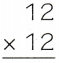Explanation: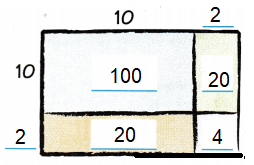10 x 10 = 100
10 x 2  =  20
2 x 10 = 20
2 x 2 = 4
100 + 20 + 20 + 4 = 144

Question 3.Explanation: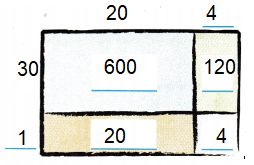30 x 20 = 600
20 x 1 = 20
30 x 4 = 120
1 x 4 = 4
600 + 120 + 20 + 4 =744

Question 4.Explanation: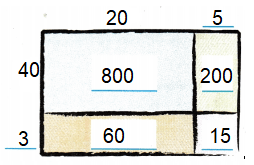800 + 200 + 60 + 15 = 1075

Question 5.Explanation:600 + 180 + 140 + 42 = 962

Math Talk

Mathematical Processes
Explain how to model and record 74 × 25.
Explanation:Problem Solving

Practice: Copy and Solve Record the product.

Question 6.
38 × 47
Explanation: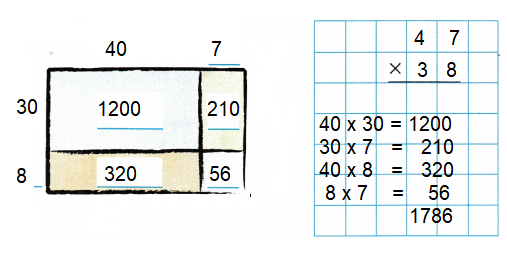Question 7.
46 × 27
Explanation: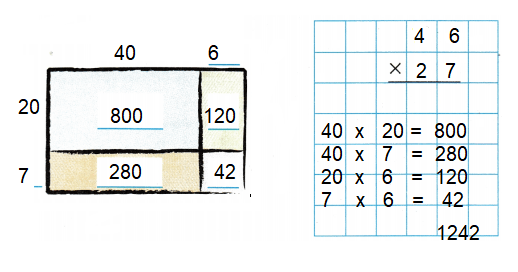Question 8.
72 × 53
Explanation:Question 9.
98 × 69
Explanation:Question 10.
53 × 68
Explanation: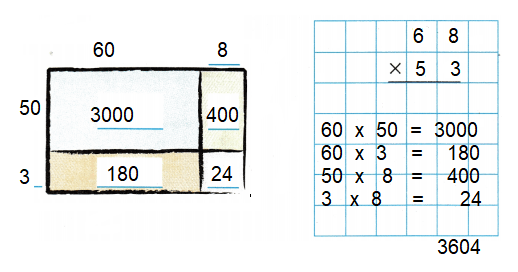Question 11.
76 × 84
Explanation: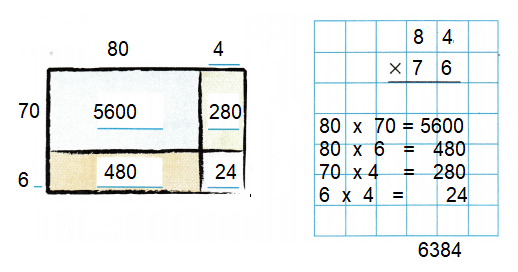Question 12.
92 × 48
Explanation: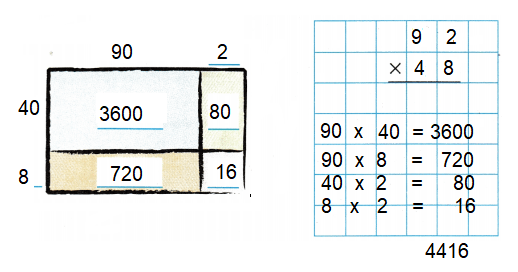Question 13.
37 × 79
Explanation:H.O.T. Algebra Find the unknown digits. Complete the problem.

Question 14.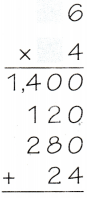76 x 24 = 1824
Explanation: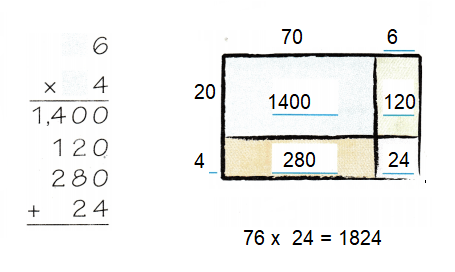Question 15.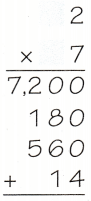82 x 97 = 7954
Explanation: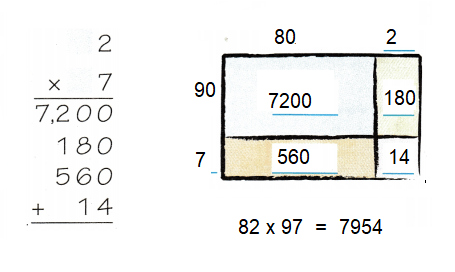Question 16.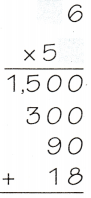36 x 53 = 1908
Explanation:Question 17.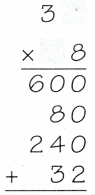34 x 28 = 952
Explanation: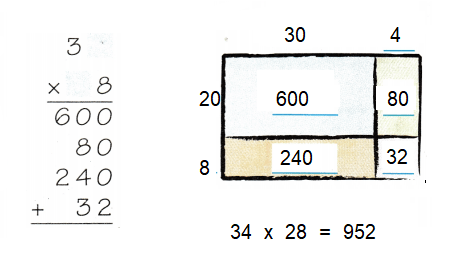Problem Solving

Use the pictograph for 18-20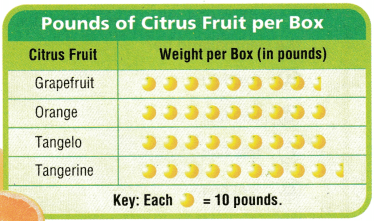Question 18.
Use Graphs A fruit-packing warehouse is shipping 15 boxes of grapefruit to a store in St. Louis, Missouri. What is the total weight of the shipment?
1,275 pounds.
Explanation:
A fruit-packing warehouse is shipping 15 boxes of grapefruit to a store.
1 box = 10 x 8.5 = 1,275 pounds.
The total weight of the shipment
15 x 85 = 150

Question 19.
How much less do 13 boxes of tangelos weigh than 18 boxes of tangerines?
Tangelos weighs 540 pounds less than Tangerines.
Explanation:
1 box of tangelos contain 9 fruits.
Weight of 1 box tangelos = 9 x 10 = 90 pounds
Weight of 13 boxes tangelos = 13 x 90 = 1,170 pounds
1 box of tangerines contain 9.5 fruits.
Weight of 1 box tangerines = 9.5 x 10 = 95 pounds
Weight of 18 boxes of tangerines = 18 x 95 = 1,710
tangerines – tangelos = 1,710 – 1,170 = 540
So, tangelos weighs 540 pounds less than tangerines.

Question 20.
What is the weight of 12 boxes of oranges?
1,080 pounds
Explanation:
Each box of oranges contain 9 fruits.
1 box of orange weighs = 9 x 10 = 90 pounds
Weight of 12 boxes of oranges = 90 x 12 = 1,080 pounds.

Question 21.
H.O.T. Each person in the United States eats about 65 fresh apples each year. Based on this estimate, how many apples do 3 families of 4 eat each year?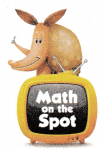780 apples.
Explanation:
Each person in the United States eats about 65 fresh apples each year.
Based on this estimate, number of apples do 3 families of 4 eat each year
3 x 4 = 12 members
Each person eats 65 apples.
So, 12 members eats = 12 x 65 = 780 apples

Question 22.
H.O.T. Multi-Step Martin has 14 packages to ship. Each package weighs 11 pounds. After shipping those 14 packages, he shipped 13 other packages that each weighed 27 pounds. How many pounds of packages did Martin ship? Explain.
505 pounds.
Explanation:
Martin has 14 packages to ship.
Each package weighs 11 pounds.
14 x 11 = 154 pounds
After he shipped 13 other packages that each weighed 27 pounds.
13 x 27 = 351 pounds
Total pounds of packages Martin shipped
154 + 351 = 505 pounds.

Question 23.
Use Math Language The product of 26 × 93 is more than 25 × 93. How much more? Explain how you know without multiplying.
26 is 1 more than 25,
So, the difference is 93.
Explanation:
The product of 26 x 93 = 2,418
The product of 25 x 93 = 2,325
The difference between these two products = 93

Question 24.
A builder is clearing more land. He clears one strip that is 32 feet wide. He needs 28 of these strips cleared. How many feet wide will the cleared land be?
(A) 840 feet
(B) 880 feet
(C) 856 feet
(D) 896 feet
Option(D)
Explanation:
A builder clears one strip that is 32 feet wide.
He needs 28 of these strips cleared.
Total feet wide the cleared land be
32 x 28 = 896 feet

Question 25.
Students line up in equal rows for a school picture. There are 41 rows of 16 students. How many students are in the school picture?
(A) 640 students
(B) 656 students
(C) 650 students
(D) 646 students
Option(B)
Explanation:
There are 41 rows of 16 students.
Number of students in the school picture
41 x 16 = 656 students

Question 26.
Multi-Step Mrs. Parker sells 18 boxes of 45 oranges at the Farmers’ Market. She sells 16 boxes of 52 lemons. How many more lemons than oranges does Mrs. Parker sell?
(A) 22 more lemons
(B) 50 more lemons
(C) 42 more lemons
(D) 20 more lemons
Option(A)
Explanation:
Mrs. Parker sells 18 boxes of 45 oranges at the Farmers’ Market.
Total oranges 18 x 45 = 810
She sells 16 boxes of 52 lemons.
Total lemons 16 x 52 = 832
832 – 810 = 22
22 lemons are more than oranges.

TEXAS Test Prep

Question 27.
Each row of apple trees has 14 trees. There are 16 rows. How many apple trees are there?
(A) 1,340
(B) 224
(C) 184
(D) 124
Option(B)
Explanation:
Each row of apple trees has 14 trees
There are 16 rows.
Number of apple trees 14 x 16 = 224

### Texas Go Math Grade 4 Lesson 8.4 Homework and Practice Answer Key

Record the product.

Question 1.Explanation: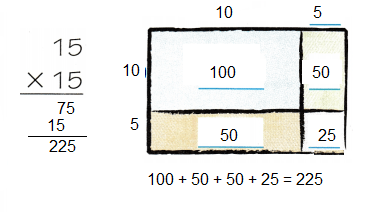Question 2.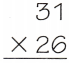Explanation: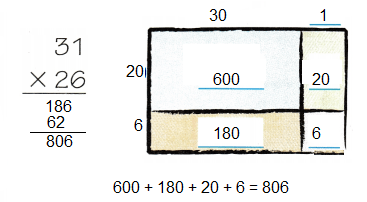Question 3.Explanation: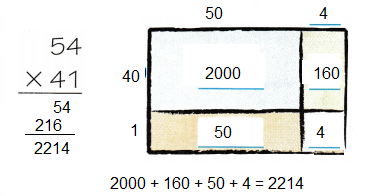Question 4.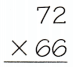Explanation: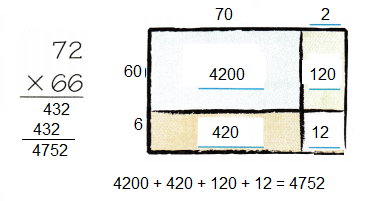Find the unknown digits. Complete the problem.

Question 5.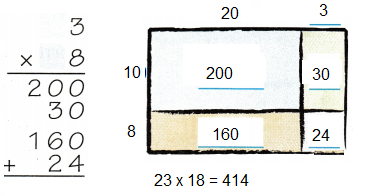Question 6.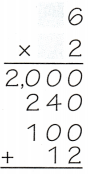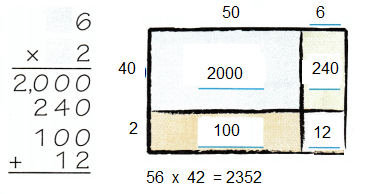Question 7.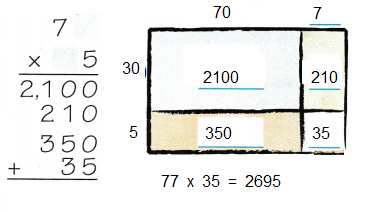Question 8.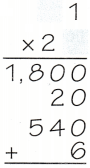Problem Solving

Use the pictograph for 9 – 12.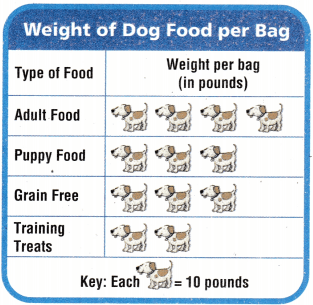Question 9.
Pet Center ordered 75 bags of training treats. How many pounds did Pet Center order?
Explanation:
Pet Center ordered 75 bags of training treats.
Each bag contain 2 training treats and each weighs10 pounds.
So, 2 x 10 = 20 pounds
Total pounds Pet Center order
75 x 20 = 1,500 pounds
Question 10.
What is the weight of 45 bags of grain free dog food?
Explanation:
Grain free bag has 3 dogs food and each weighs 10 pounds.
So, 3 x 10 = 30 pounds.
The weight of 45 bags of grain free dog food = 45 x 30 = 1,350 pounds

Question 11.
The animal shelter bought 28 bags of puppy food and 36 bags of adult dog food. What is the total weight of their order?
Explanation:
The animal shelter bought 28 bags of puppy food 28 x 3 x 10 = 840 pounds
36 bags of adult dog food 36 x 4 x 10 = 1,440 pounds
Total weight of their order 1,440 + 840 = 2,280 pounds.

Question 12.
A warehouse shipped 68 bags of adult dog food and 45 bags of grain free dog food. How many more pounds of adult dog food did they ship?
Explanation:
A warehouse shipped 68 bags of adult dog food
68 x 4 x 10 = 2,720 pounds
A ware house shipped 45 bags of grain free dog food.
45 x 3 x 10 = 1,350 pounds
Number of more pounds of adult dog food they shipped
2,720 – 1,350 = 1,370 pounds

Lesson Check

Question 13.
A farmer bought 19 bags of chicken feed. Each bag costs $57. I low much does the farmer pay for the chicken feed? (A)$1064
(B) $1,074 (C)$1,023
(D) $1,083 Answer: Option (D) Explanation; A farmer bought 19 bags of chicken feed. Each bag costs$57.
Total amount the farmer paid for the chicken feed
19 x 57 = $1,083 Question 14. Visitors at a petting zoo are given food to feed the ducks. A 50-pound hag of duck food costs$71. The zoo needs 44 bags of food for the summer months. How much does the duck food cost for the summer?
(A) $3,550 (B)$2,200
(C) $3,124 (D)$2,024
Option(C)
Explanation:
A 50-pound bag of duck food costs $71. The zoo needs 44 bags of food for the summer months. Cost of the duck food for the summer 44 x 71 =$3,124

Question 15.
Large flower pots weigh 28 pounds each. One crate holds 35 pots. What is the weight of one crate of flower pots?
(A) 780 pounds
(B) 980 pounds
(C) 940 pounds
(D) 920 pounds
Option (B)
Explanation:
Large flower pots weigh 28 pounds each.
One crate holds 35 pots.
The weight of one crate of flower pots
28 x 35 = 980 pounds

Question 16.
Multi-Step A store sells drinks in cartons of 6 each. Bonnie bought 2 cartons of lemonade and 2 cartons of fruit juice. Each bottle is 32 ounces. How many ounces did Bonnie buy?
(A) 560 ounces
(B) 42 ounces
(C) 840 ounces
(D) 768 ounces
Option(D)
Explanation:
A store sells drinks in cartons of 6 each.
Bonnie bought 2 cartons of lemonade and 2 cartons of fruit juice.
Total cartons he bought 2 + 2 = 4
Each bottle is 32 ounces.
Each carton has 6 bottles = 32 x 6 = 192 ounces
Number of ounces Bonnie bought in all
192 x 4 = 768 ounces

Question 17.
Multi-Step Twelve adults and 63 students are going on a field trip to the aquarium. Tickets cost $18 for adults and$15 for students. What is the total cost of the tickets?
(A) $1,350 (B)$1,125
(C) $1,161 (D)$1,314
Option(C)
Explanation:
Twelve adults and 63 students are going on a field trip to the aquarium.
Tickets cost $18 for adults 12 x 18 =$216
Ticket cost $15 for students, 15 x 63 =$945
The total cost of the tickets 945 + 216 = $1,161 Question 18. Multi-Step A bus company sold 39 one-way tickets and 20 round-trip tickets from West Elmwood to East Elmwood. One-way tickets cost$14. Round trip tickets cost $25. How much money did the bus company collect? (A)$1,046
(B) S1,255
(C) $916 (D)$946
One-way tickets cost $14; 39 x 14 =$546
Round trip tickets cost $25; 20 x 25 =$500
$546 +$500 = \$946